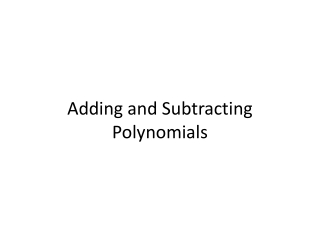DownloadDownload PresentationTélécharger la présentation- - - - - - - - - - - - - - - - - - - - - - - - - - - E N D - - - - - - - - - - - - - - - - - - - - - - - - - - -
Presentation Transcript

2. 1. Determine whether the given expression is a monomial (Yes or No). For those that are monomials, state the coefficient and degree. (similar to p.323 #30)

3. 2. Determine whether the given expression is a monomial (Yes or No). For those that are monomials, state the coefficient and degree. (similar to p.323 #32)

4. 3. Determine whether the given expression is a monomial (Yes or No). For those that are monomials, state the coefficient and degree. (similar to p.323 #34)

5. 4. Determine whether the given expression is a monomial (Yes or No). For those that are monomials, state the coefficient and degree. (similar to p.323 #38)

6. 5. Determine whether the given expression is a monomial (Yes or No). For those that are monomials, state the coefficient and degree. (similar to p.323 #40)

7. 6. Determine whether the given expression is a polynomial (Yes or No). If it is a polynomial, write the polynomial in standard form, determine its degree, and state if it is a monomial, binomial, or trinomial. If it is a polynomial with more than 3 terms, identify the expression as a polynomial (similar to p.323 #42)

8. 7. Determine whether the given expression is a polynomial (Yes or No). If it is a polynomial, write the polynomial in standard form, determine its degree, and state if it is a monomial, binomial, or trinomial. If it is a polynomial with more than 3 terms, identify the expression as a polynomial (similar to p.323 #44)

9. 8. Determine whether the given expression is a polynomial (Yes or No). If it is a polynomial, write the polynomial in standard form, determine its degree, and state if it is a monomial, binomial, or trinomial. If it is a polynomial with more than 3 terms, identify the expression as a polynomial (similar to p.323 #46)

10. 9. Determine whether the given expression is a polynomial (Yes or No). If it is a polynomial, write the polynomial in standard form, determine its degree, and state if it is a monomial, binomial, or trinomial. If it is a polynomial with more than 3 terms, identify the expression as a polynomial (similar to p.323 #50)

11. 10. Determine whether the given expression is a polynomial (Yes or No). If it is a polynomial, write the polynomial in standard form, determine its degree, and state if it is a monomial, binomial, or trinomial. If it is a polynomial with more than 3 terms, identify the expression as a polynomial (similar to p.323 #52)

12. 11. Determine whether the given expression is a polynomial (Yes or No). If it is a polynomial, write the polynomial in standard form, determine its degree, and state if it is a monomial, binomial, or trinomial. If it is a polynomial with more than 3 terms, identify the expression as a polynomial (similar to p.323 #54)